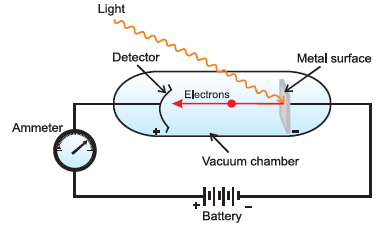# Photoelectric effect

## Photoelectric Effect

It was discovered by Hertz.
When a beam of light of certain frequency (threshold frequency) strikes the metal surface, electrons are emitted or ejected from the metal surface. this phenomenon is known as photoelectric effect.
Here is Equipment for studying the photoelectric effect.Light of a particular frequency strikes a clean metal surface inside a vacuum chamber. Electrons are ejected from the metal and are counted by a detector that measures their kinetic energy.

### Observations in Photoelectric Effect

(1)For each metal there is a characteristic minimum frequency below which photoelectric effect is not observed. This is called threshold frequency.
If frequency of light is less than the threshold frequency there is no ejection of electrons no matter how long it falls on surface or how high is its intensity
(2) The kinetic Energy of electrons emitted is directly proportional to frequency of striking photons & independent of their intensity
(3) The no. of electrons that are ejected per second from metal surface depends upon intensity of striking radiations and doesn’t depend upon their frequency.

### Explanation of Photoelectric Effect

Einstein could explain photoelectric effect using Plank’s Quantum theory as follows: -
(a) Photoelectrons are ejected only when incident light has threshold frequency. ( $h \nu - h \nu _0$)
(b) If frequency of incident light is more than threshold frequency then the excess energy is imparted to electrons in the form of kinetic energy. ($E - W =\frac {1}{2} mv^2$)
$E = W + \frac {1}{2} mv^2$
$h \nu = h \nu _0 + \frac {1}{2} mv^2$
(c) Greater he frequency of incident light, greater the kinetic energy of e-.
(d) Greater the intensity of light more the no. of electrons ejected.

Question 1
Electrons are emitted with zero velocities from metal surface when exposed to radiation of wavelength 6800 Ao. Calculate $\nu _0$ & $W_0$ ?
Solution
Given $\lambda = 6800 \; A^0 = 6800 \times 10^{-10} \; m = 6.8 \times 10^{-7}$ m
$c = 3 \times 10^{8}$ m/s

Also $\lambda = \frac {c}{\nu}$ or $\nu = \frac {c}{\lambda}$
Therefore putting the values in the equation, we get

$=\frac {3 \times 10^8}{ 6.8 \times 10^{-7}} = 4.41 \times 10^{14}$ \sec
Hence, work function (W0) of the metal $= h \nu _0$
$= (6.626 \times 10^{-34}) (4.41 \times 10^{14})$
$= 2.922 \times 10^{-19}$ J

Question 2
When electromagnetic radiation of wavelength 300 nm falls on the surface of sodium, electrons are emitted with a kinetic energy of $1.68 \times 10^5$ J/mol. What is the minimum energy needed to remove an electron from sodium? What is the maximum wavelength that will cause a photoelectron to be emitted?
Solution
The energy (E) of a 300 nm photon is given by
$=h \nu = \frac {hc}{\lambda}$
$=\frac {6.626 \times 10^{-34} \times 3 \times 10^{8}}{300 \times 10^{-9}}$
$= 6.626 \times 10^{-19}$ J
Therefore, The energy of one mole of photons
$= 6.626 \times 10^{-19} \times 6.022 \times 10^{23}$ J/mole
$= 3.99 \times 10^{5}$ J/mole
The minimum energy needed to remove one mole of electrons from sodium
$= (3.99 -1.68) 10^5$ J/mole
$= 2.31 \times 10^5$ J/mole
The minimum energy for one electron
$=\frac {2.31 \times 10^5}{6.022 \times 10^{23}}$
$=3.84 \times 10^{-19}$ J
This corresponds to the wavelength
$\lambda = \frac {hc}{E}$
$=\frac {6.626 \times 10^{-34} \times 3 \times 10^{8}}{3.84 \times 10^{-19}}$
= 517 nm
(This corresponds to green light)

Question 3
A proton of wavelength 400 nm strikes metal surface. The electrons are ejected with velocity 5.85 × 105 m/s. Calculate min. energy required to remove electron from metal surface. (Mass of electron = 9.1 × 10-31 kg)
Solution
Given:-
λ = 400 nm
Velocity = 5.85 × 105 m/s
C = νλ

3 × 108 m/s = ν. 400 × 10(-9) m
or
ν= 7.5 × 10(14) m
Now E = hν
= 6 × 10-34 × 7.5 × 1014
= 45 ×10-20 J
Kinetic Energy of Electron =1/2 mv2
=.5 × 9.1 × 10-31 × 5.85 × 105 × 5.85 × 105
=15.57 ×10-20 J
Min Energy = 45 ×10-20 J - 15.57 ×10-20 J = 29.43 ×10-20 J